# Multiplication by 2 Digit Numbers

## NUMBERS AND OPERATIONSThe multiplication is that operation that consists of calculating the product (result) that is obtained by adding the same number (factor or multiplicand) so many times as the value of the other number (factor or multiplicator or multiplier).

Multiplication is used when we have to replace the calculation of certain repetitive additions (for example: 2 + 2 + 2 + 2 = 8) by a more efficient method (2 x 4 = 8).

In general, multiplication is considered the inverse operation of division.

#### Multiply natural numbers without carrying: 3 digits by 2 digits

We present you some educational worksheets in PDF format accompanied by their explanation and an online game so that you can learn to solve the three-digit multiplications (multiplicand) by two digits (multiplier).

SPANISHENGLISHLook at the solved multiplication that we propose and you will learn how to calculate a multiplication of three digits in the multiplicand by two digits in the multiplier without regrouping.

LET'S MULTIPLY 621 x 231st) We start by multiplying the ones of the multiplier (table of 3) from right to left and for each of the multiplicand digits:

3 x 1 = 3 ones

3 x 2 = 6 tens

3 x 6 = 18 hundreds

We will write the results below the horizontal dividing line and aligning them according to their place value from the ones.

2nd) We start a new line and write a zero to the right to align the figures from the tens, we will repeat the process of multiplying again from right to left.

3rd) We will multiply the tens of the multiplier (table of 2) by each of the digits of the multiplicand:

2 x 1 = 2 ones

2 x 2 = 4 tens

2 x 6 = 12 hundreds

We will write the results below the horizontal dividing line and aligning them according to their place value from the tens.4th) We will write a horizontal dividing line, we will draw the sign of the addition (+) and then we will add the products obtained with the previous multiplications from right to left.

Finally, we will write the result of the sum below the horizontal dividing line.

You've got it! The solution: 621 x 23 = 14,283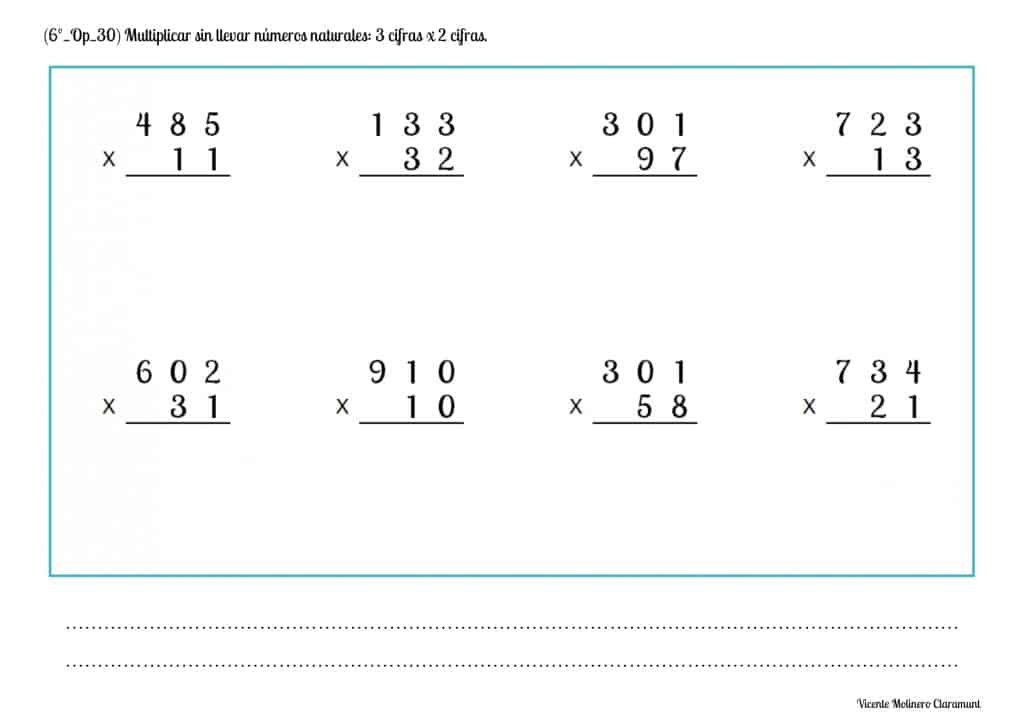#### Solve multiplication word problems without carrying with natural numbers: 3 digits x 2 digits

We offer you a series of multiplication word problems of three figures by two figures (without regrouping) for you to continue improving. We also provide you with video lessons where we explain in a simple way what is the procedure for its resolution. Time to practice!

SPANISHENGLISH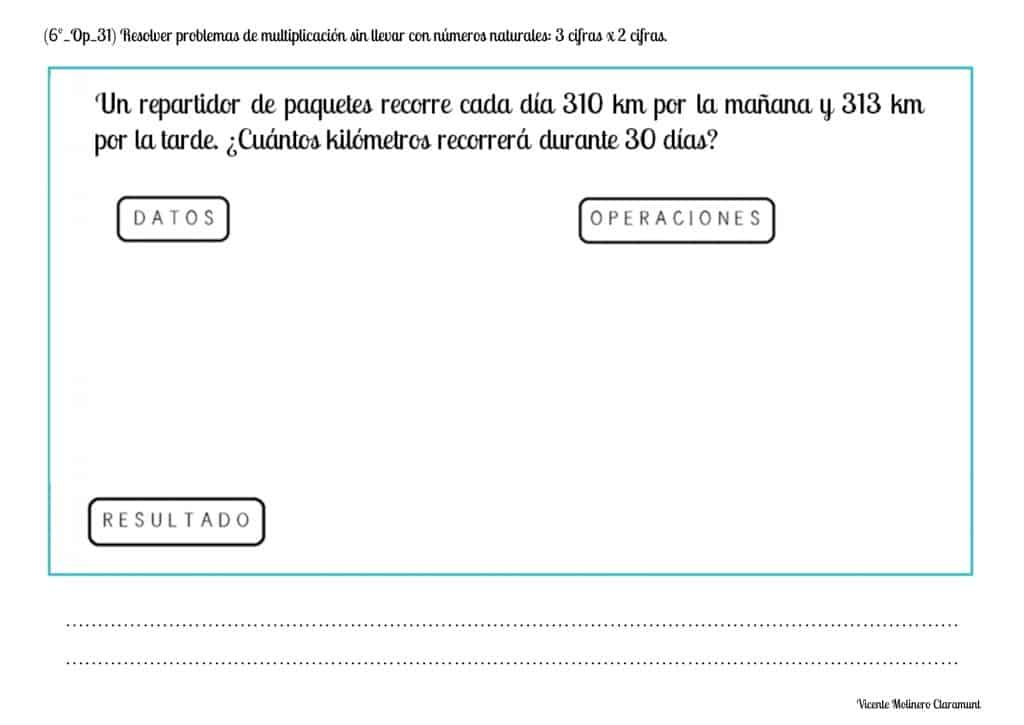#### Multiply natural numbers with carrying: 3 digits by 2 digits

We present you some downloadable and printable exercises accompanied by their pedagogical lesson and an interactive activity so that you can learn to easily solve the multiplications by carrying of three-digits (multiplicand) by two-digits (multiplier).

SPANISHENGLISHWe show you a solved example that will help you learn step by step how to solve three-digits multiplication in the multiplicand by two-digits in the multiplier and regrouping.

LET'S MULTIPLY 472 x 56

1st) We start by multiplying the ones of the multiplier (table of 6) from right to left and for each of the multiplicand digits.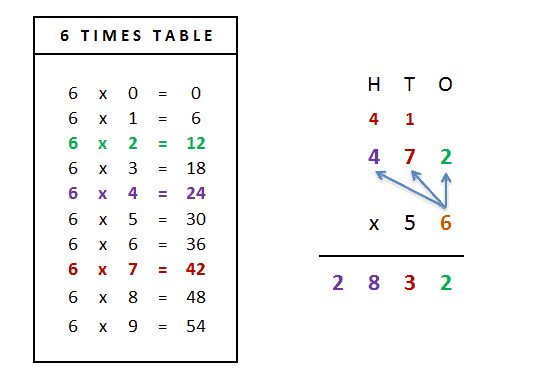If the result of the multiplication of two digits is equal to or greater than 10 (for example, 8 x 4 = 32), we will move the digit on the left (in this example it would be 3) to the next column to add it to the result of the next multiplied number.

6 x 2 = 12 ones, that is, 1 ten and 2 ones

6 x 7 = 42 tens + 1 ten = 43 tens, that is, 4 hundreds and 3 tens

6 x 4 = 24 hundreds + 4 hundreds = 28 hundreds

2nd) We start a new line and write a zero to the right to align the figures from the tens, we will repeat the process of multiplying again from right to left.

3rd) We will multiply the tens of the multiplier (table of 5) by each of the digits of the multiplicand.

Remember, if the result of each multiplication is greater than or equal to 10, we will move the digit on the left to the next column to add it to the result of the next multiplication.

5 x 2 = 10 ones, that is, 1 ten and 0 ones

5 x 7 = 35 tens + 1 ten = 36 tens, that is, 3 hundreds and 6 tens

5 x 4 = 20 hundreds + 3 hundreds = 23 hundreds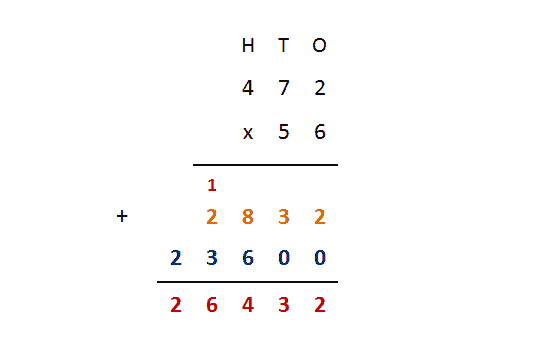4th) We will write a horizontal dividing line, we will draw the sign of the addition (+) and then we will add the products obtained with the previous multiplications from right to left.

Finally, we will write the result of the sum below the horizontal dividing line.

You've got it! The solution: 472 x 56 = 26,432#### Solve multiplication word problems with carrying with natural numbers: 3 digits x 2 digits

We propose you our multiplication word problems of three figures by one figure (regrouping) so that you continue to improve. In addition, we provide you with video lessons where we explain in a practical way what is the procedure for its correct resolution.

SPANISHENGLISH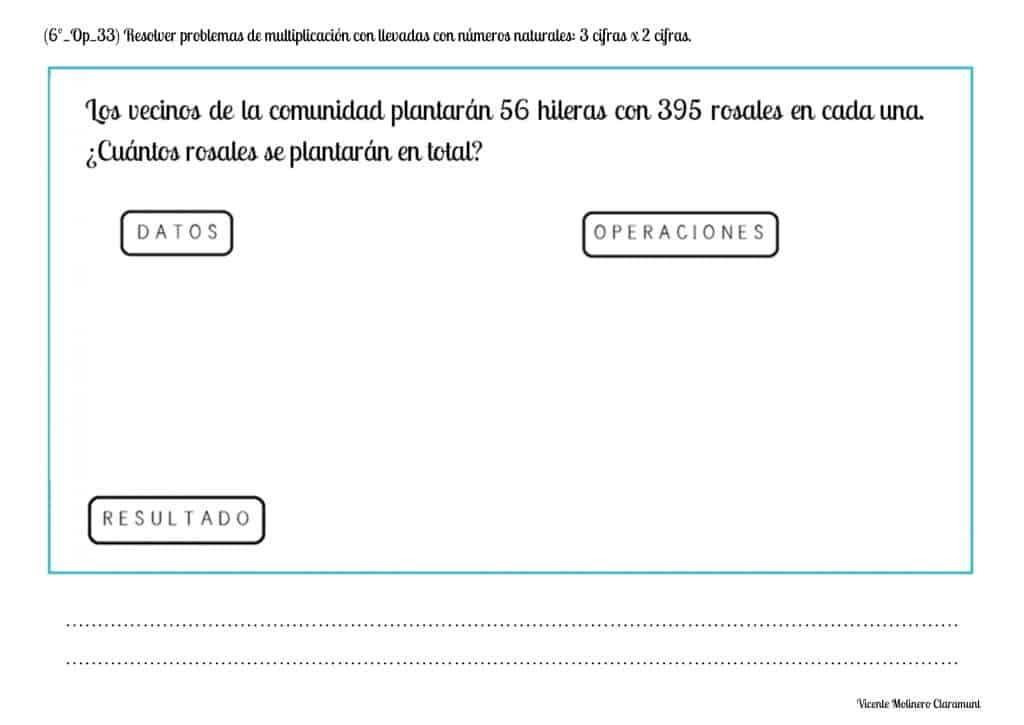#### Multiply natural numbers without carrying: 4 digits by 2 digits

We present some educational activities with the numbers up to 9,999 in the multiplicand together with their explanation so that you can quickly learn how to find multiplications without regrouping of four digits by two digits.

SPANISHENGLISHLook at the practical example that we provide you with using a multiplication without carrying of 4-digits in the multiplicand and 2-digits in the multiplier.

LET'S MULTIPLY 4,213 x 32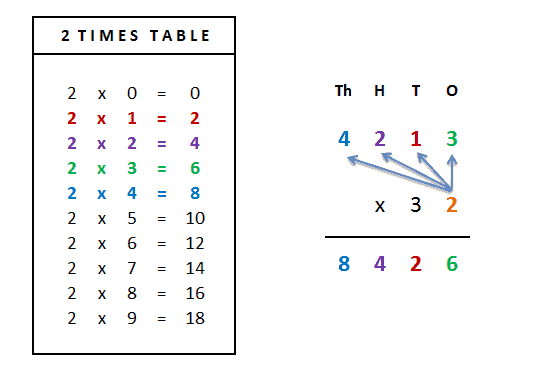1st) We start by multiplying the ones of the multiplier (table of 2) from right to left and for each of the multiplicand digits:

2 x 3 = 6 ones

2 x 1 = 2 tens

2 x 2 = 4 hundreds

2 x 4 = 8 thousands

We will write the results below the horizontal dividing line and aligning them according to their place value from the ones.

2nd) We start a new line and write a zero to the right to align the figures from the tens, we will repeat the process of multiplying again from right to left.

3rd) We will multiply the tens of the multiplier (table of 3) by each of the digits of the multiplicand:

3 x 3 = 9 ones

3 x 1 = 3 tens

3 x 2 = 6 hundreds

3 x 4 = 12 thousands

We will write the results below the horizontal dividing line and aligning them according to their place value from the tens.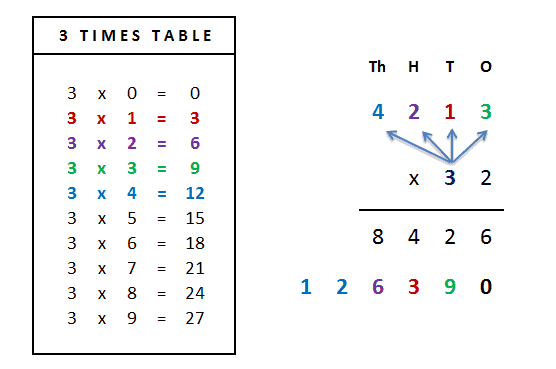4th) We will write a horizontal dividing line, we will draw the sign of the addition (+) and then we will add the products obtained with the previous multiplications from right to left.

Finally, we will write the result of the sum below the horizontal dividing line.

You've got it! The solution: 4,213 x 32 = 134,816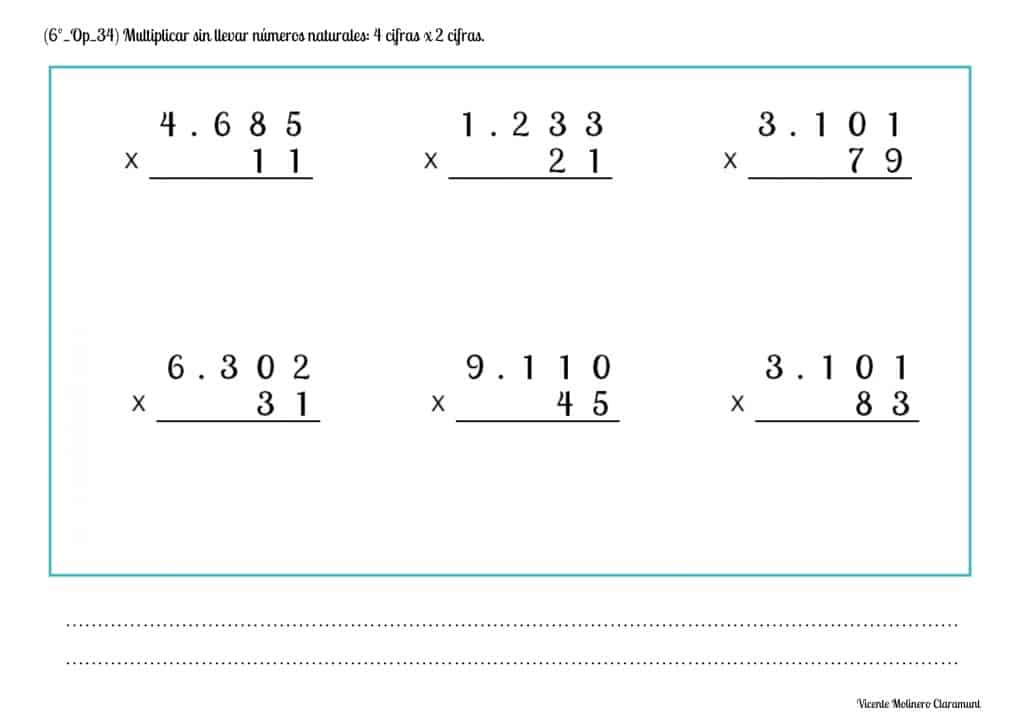#### Solve multiplication word problems without carrying with natural numbers: 4 digits x 2 digits

We provide you with a variety of multiplication word problems of four figures by two figures (without regrouping) so that you can continue to improve your calculation skills and solving word problem situations.

SPANISHENGLISH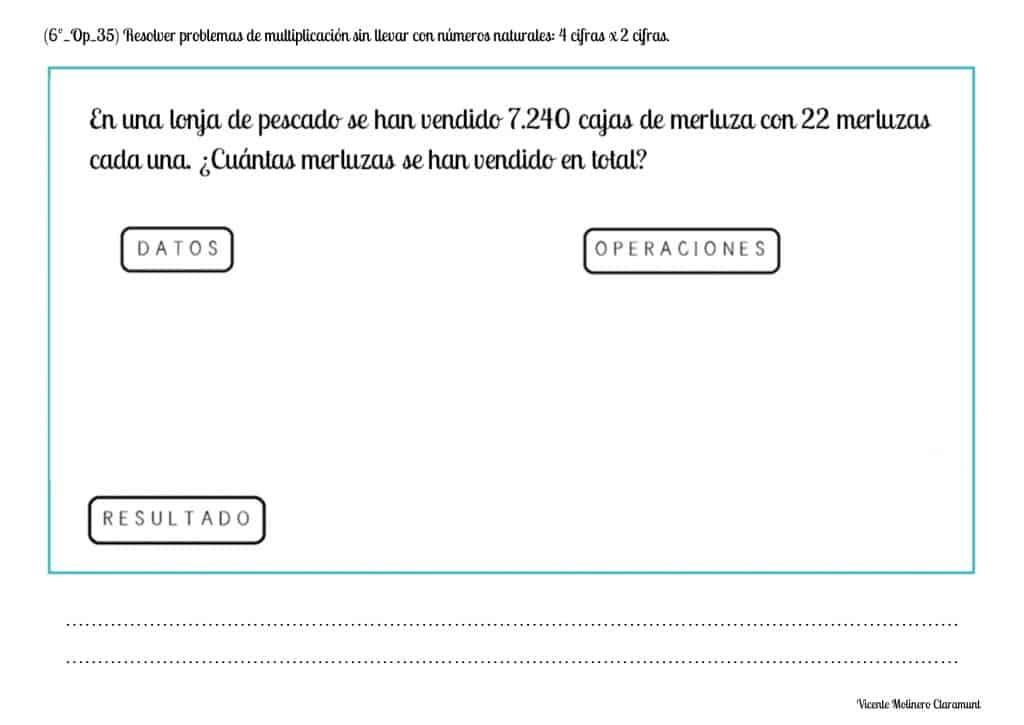#### Multiply natural numbers with carrying: 4 digits by 2 digits

We offer you pedagogical exercises prepared for downloading and printing in PDF supported by a didactic tutorial so that you can quickly understand how to find the multiplications by carry over of four digits (multiplicand) by two digits (multiplier).

SPANISHENGLISHWe show you a solved example that will help you learn step by step how to solve 4-digits multiplication in the multiplicand by 2-digits in the multiplier and regrouping.

LET'S MULTIPLY 3,956 x 97

1st) We start by multiplying the ones of the multiplier (table of 7) from right to left and for each of the multiplicand digits:

If the result of the multiplication of two digits is equal to or greater than 10 (for example, 8 x 4 = 32), we will move the digit on the left (in this example it would be 3) to the next column to add it to the result of the next multiplied number.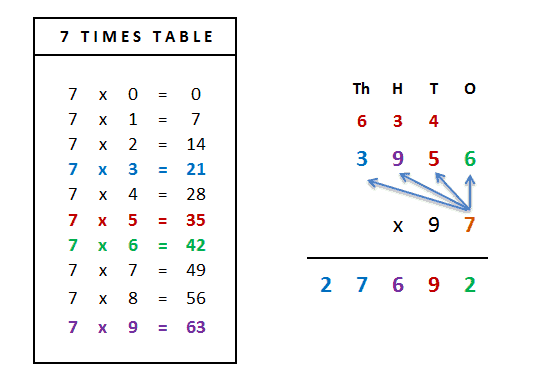7 x 6 = 42 ones, that is, 4 tens and 2 ones

7 x 5 = 35 tens + 4 tens = 39 tens, that is, 3 hundreds and 9 tens

7 x 9 = 63 hundreds + 3 hundreds = 66 hundreds, that is, 6 thousands and 6 hundreds

7 x 3 = 21 thousands + 6 thousands = 27 thousands

2nd) We start a new line and write a zero to the right to align the figures from the tens, we will repeat the process of multiplying again from right to left.

3rd) We will multiply the tens of the multiplier (table of 9) by each of the digits of the multiplicand.

Remember, if the result of each multiplication is greater than or equal to 10, we will move the digit on the left to the next column to add it to the result of the next multiplication.

9 x 6 = 54 ones, that is, 5 tens and 4 ones

9 x 5 = 45 tens + 5 tens = 50 tens, that is, 5 hundreds and 0 tens

9 x 9 = 81 hundreds + 5 hundreds = 86 hundreds, that is, 8 thousands and 6 hundreds

9 x 3 = 27 thousands + 8 thousands = 35 thousands4th) We will write a horizontal dividing line, we will draw the sign of the addition (+) and then we will add the products obtained with the previous multiplications from right to left.

Finally, we will write the result of the sum below the horizontal dividing line.

You've got it! The solution: 3,956 x 97 = 383,732#### Solve multiplication word problems with carrying with natural numbers: 4 digits x 2 digits

We present you various multiplication word problems of four figures by two figures (regrouping) so that you can continue developing your calculation skills, your logical-mathematical reasoning and the resolution of problems.

SPANISHENGLISH#### Multiply natural numbers by 10, 100, 1,000 and so on

Do you know how to multiply a number by the unit followed by zeros? We will explain it to you below!

First, watch the video explanation and learn with simple steps to multiply any natural number by the unit followed by zeros: 10, 100, 1,000, 10,000, 100,000 and so on.

SPANISHENGLISHThe procedure is very simple: only as many zeros are added to the end as there are in the number we have multiplied by, as shown in the examples.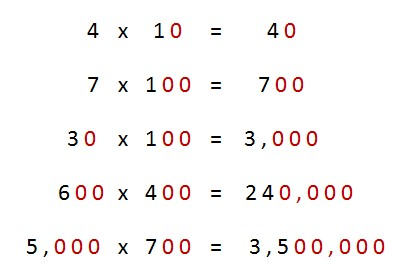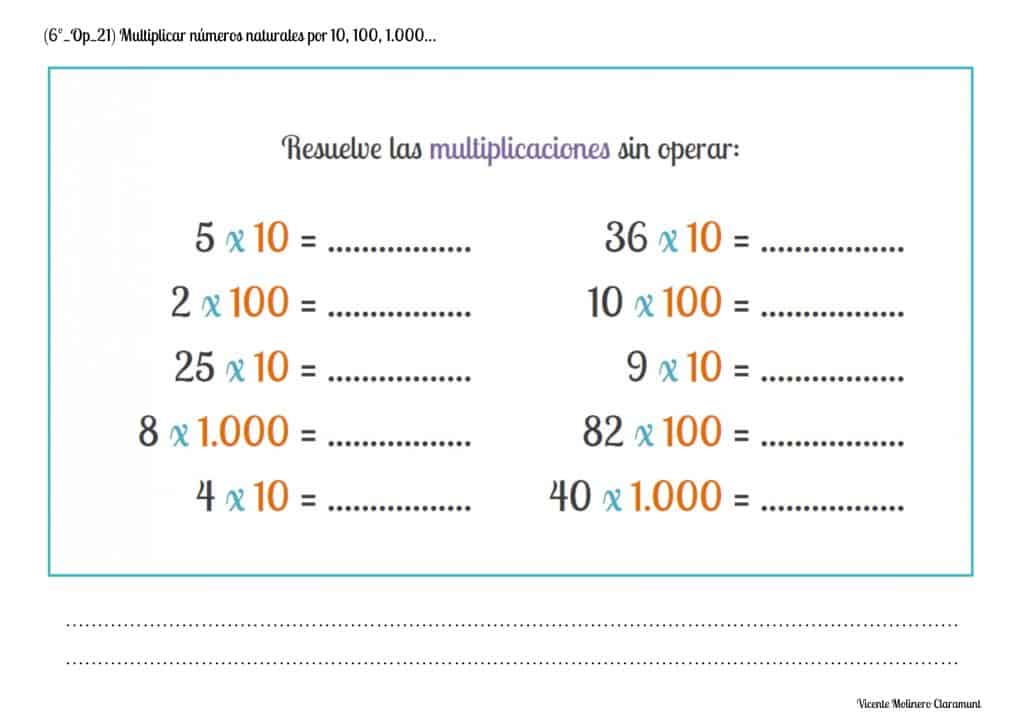#### Mentally calculate simple operations: Multiplication

Here are some mental math activities to test your memory and show that you already know all the multiplication tables.#### Applying the Commutative Property of Multiplication

One of the most important fundamental properties of multiplication is "commutativity":

A x B = B x A

The Commutative Property of Multiplication states that "the order in which the factors are multiplied does not alter the product".

In other words, when we multiply two numbers (factors) with each other, the order in which they are multiplied will not matter because the result (product) will always be the same.

SPANISHENGLISH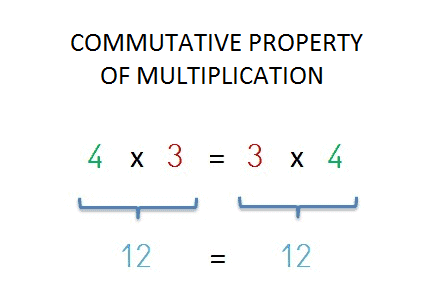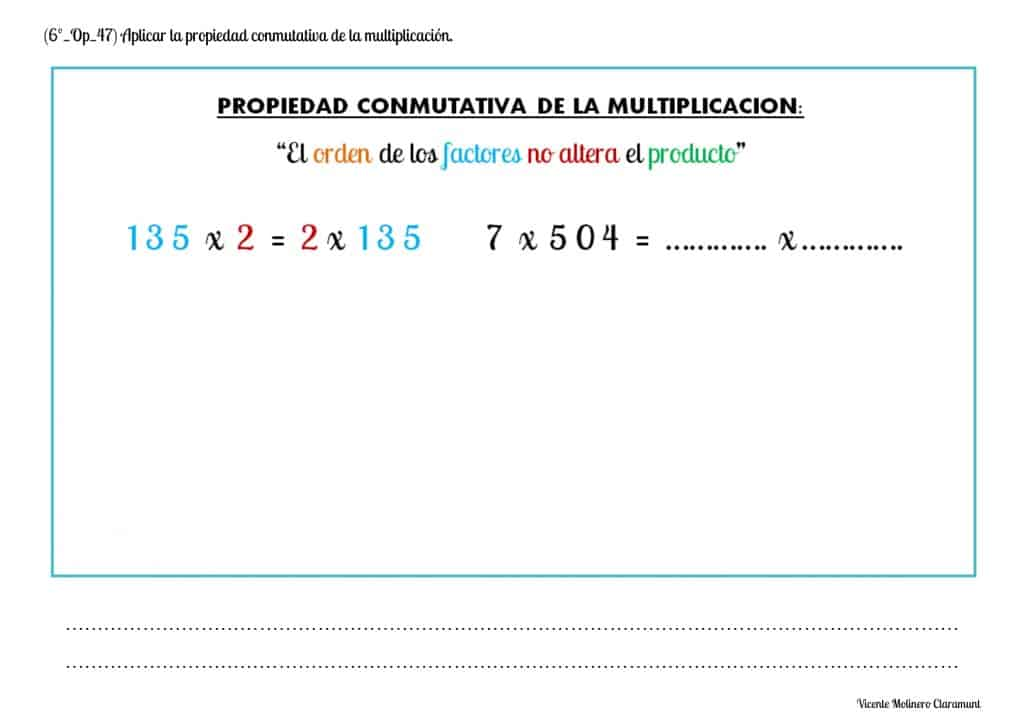#### Apply the Associative Property of Multiplication

Another of the fundamental properties of multiplication is "associativity", where the use of parentheses tells us in what order we must solve the multiplications :

A x ( B x C ) = ( A x B ) x C

The Associative Property of Multiplication determines that "when three or more numbers are multiplied, the result is the same regardless of the order in which the factors are multiplied".

In other words, when we multiply three or more numbers (factors) with each other, the order in which they are multiplied will not matter because the result (product) will always be the same.

SPANISHENGLISH#### Apply the Distributive Property of Multiplication over Addition

We are going to learn yet another one of the fundamental properties of multiplication, in this case, the property called "distributivity of addition", where both the addition and multiplication algorithms are combined through the use of parentheses, which indicate the hierarchy or order in which we must solve the combined operations:

A x ( B + C ) = ( A x B ) + ( A x C )

The Distributive Property of Multiplication over addition states that " the sum of two numbers multiplied by a third number is equal to the sum of each addend multiplied by the third number".

SPANISHENGLISH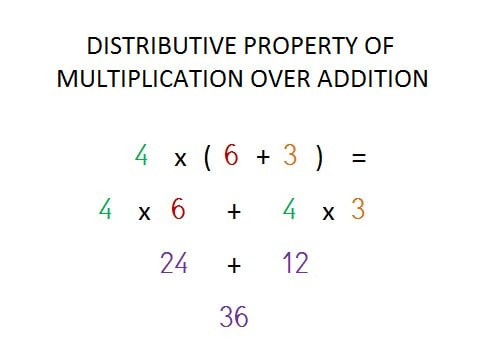#### Apply the Distributive Property of Multiplication over Subtraction

Finally, we will learn the fourth of the fundamental properties of multiplication, the property known as the "distributivity of subtraction", where both the subtraction and multiplication algorithms are combined through the use of parentheses, which indicate the hierarchy or order in which we must solve the combined operations:

A x ( B - C ) = ( A x B ) - ( A x C )

The Distributive Property of Multiplication over subtraction establishes that "the subtraction of two numbers multiplied by a third number is equal to the subtraction of each number multiplied by the third number".

SPANISHENGLISHTell me and I forget, teach me and I remember, involve me and I learn

Benjamin Franklin